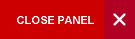## Electric Circuit Analysis

Semester 1

This course introduces students to the fundamental building blocks of electrical and electronic circuit theory. The basic electrical circuit tools required to analyze the characteristics, the behaviour, functionality and performance characteristics of electrical circuits containing resistors, inductors and capacitors are introduced. These tools are applied to obtain both the full dynamic performance of circuits and the steady state performance of sinusoidal systems.

Syllabus:
• Concepts in basic electrical quantities: electronic charge, current, voltage, power, energy
• Introduction to circuit theory
• Simple circuits
• Kirchhoff’s voltage and current laws
• Series and parallel circuit networks
• Structured Circuit Theory
• Network theorems: Superposition, Thevenin’s, Norton’s
• Solution using structured approach
• Network analysis: branch, loop, node
• Source types
• Maximum power transfer theorem
• Capacitive and inductive circuits
• Laplace models
• Steady state and dynamic responses of simple networks
• Circuit Theory in Laplace domain
• Transient and steady state solutions Complex number models
• Complex power
• Power factor correction
Evaluation:

One 2-hour theory final exam paper – 60%

Mid Semester exam -   20%

Assignments                 20%

• Six take-home problem solving assignment of equal weighting (10%)
• One paper on a survey of the state-of-the-art in the analogue circuit designs (10%). Report will take the form of that required for an IEEE paper publication.
Learning Objectives:

Upon successful completion of this course, students should be able to:

• Use basic graph theory and Kirchhoff’s Laws to solve linear RLC electric circuits directly and via circuit reduction techniques
• Apply mesh analysis and nodal analysis techniques to solve for currents and voltages in DC circuits
• Use Thevenin’s and Norton Theorems to analyze DC circuits
• Determine transient, initial condition and steady state solutions of linear RLC electric circuits using Laplace Transform
• Apply the principles of linearity and superposition
• Determine steady state solution of linear RLC electric circuit with sinusoidal inputs using the complex number model
• Perform power calculations for linear RLC circuits with sinusoidal inputs using the concept of complex power and its use in power factor correction
P14A/(PHYS1411 and PHYS1412) and P14B/(PHYS1421 and PHYS1422) and ELET1400 and M08B/MATH0100, M08C/MATH0110 or Equivalent

Text book:

Basic Engineering Circuit Analysis, 9th Edition; by J. David Irwin, R. Mark Nelms, Jan 2008